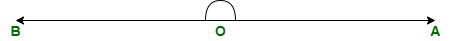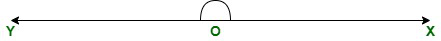# What is an angle of 180° called?

• Last Updated : 17 Aug, 2021

Geometry has been an essential part of the development of the modern world as it is applicable to designing, constructional works, architecture in choice of material for construction, and many more. It is also a vital part of the technical background for computing various designs, manufacturing, creating blueprints, programming, etc.

Geometry is a branch of mathematics that deals with the study of shapes and their properties.

Attention reader! All those who say programming isn't for kids, just haven't met the right mentors yet. Join the  Demo Class for First Step to Coding Coursespecifically designed for students of class 8 to 12.

The students will get to learn more about the world of programming in these free classes which will definitely help them in making a wise career choice in the future.

The approach for geometry can be observed from ancient times in their constructions from the use of various shapes in a very specific way. The term is originally derived from Greek words ‘ge’ and ‘materia’ which means earth and measurement respectively.

The given article is a study about angles, their types, and properties mainly focused on straight angles or 180° angles.

### What are Angles?

Angles are the space between two intersecting lines meeting at a certain point

They consist of two arms known as sides of the angle and a meeting point where angle forms called a vertex. Angles are measured in degrees which measures from 0° to 360°.

Angles are divided into different types on the basis of measurement and rotation.

### Types of Angles

Angles on the basis of measurement:

• Acute angle: The angle that measures less than 90° is the acute angle.
• Right angle: The angle that exactly measures 90° is a right angle.
• Obtuse angle: The angle that measures more than 90° and less than 180° is an obtuse angle.
• Straight angle: The angle that measures exactly 180° is a straight angle. Straight angles form straight lines.
• Reflex angle: The angle that measures more than 180° and less than 360° is the reflex angle.

Angles on the basis of rotation:

• Positive angles: The angle that moves anticlockwise from its base and is drawn from the point (x, y) is a positive angle.
• Negative angles: The angle that moves clockwise from its base and is drawn from the point (-x, -y) is a negative angle.

### What is an angle of 180° called?

180 angles widely known as straight angles are the angles that exactly measures 180°. Straight angles have two arms pointing towards opposite directions. A straight angle is also measured as(pi) angle.

It is a straight line with two endpoints where one arm moves to the opposite direction from the vertex. Straight angles can also be taken as the sum of two right angles that is 90° + 90° = 180°

The measure of straight angle can be positive or negative. If we move  anticlockwise the straight angle will measure 180° and if we move clockwise the angle will measure -180°In the above-shown figure of a straight angle, we can observe that O is the meeting point of two arms that are called vertex and OA and OB are two sides of the angle.

### Construction of a Straight Angle

Let’s look into a step-by-step construction of a straight angle.

Step 1: Draw a straight-line OX in which O will be the vertex of the angle.

Step 2: Take a protector and place its baseline on the line where point A should be placed at 0°. And follow the protector towards 180° to mark point Y.

Step 3: Join the points O and B so that another arm of the angle would form pointing in the opposite direction of OA.### Properties of Straight Angle

• A straight angle can be formed by the summation of two right angles that is 90° + 90° = 180°
• Both arms of a straight angle point in the opposite direction.
• It completes half of the rotation.  As 180° is half of 360°.

### Sample Questions

Question 1: Can we say that a triangle is a straight angle?

No, because as we know that a triangle is an enclosed figure with three sides joining together and a straight angle forms a straight line that measures 180°. Hence, both of them are different.

Question 2: Is all straight lines a straight angle?

The straight lines with two endpoints facing in the opposite direction from the vertex is a straight angle.

Question 3: Can a straight line be a reflex angle?